Next: Linear Compton Scattering Up: Laser Previous: Laser

### Laser Geometry

Define a coordinate system attached to a laser. Let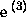be the unit vector along the direction of propagation, and introduce a unit vector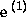perpendicular toand another unit vector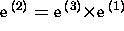. The three vectors (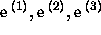) form an orthonormal frame. Define the components of these vectors in the original frame (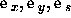) as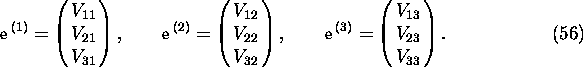Then,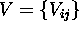is a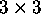orthogonal matrix. Let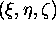be the spatial coordinate in this frame. Define the origin of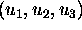as the laser focus and let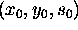be its coordinate in the original frame and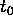the time when the laser pulse center passes the origin. Introduce a time coordinate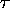whose origin is. Now, the relation between (t,x,y,s) and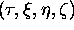is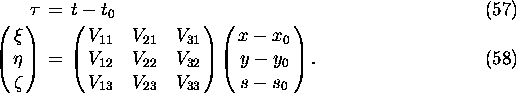A plane wave is written in the form, in thecoordinate,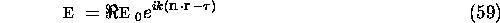where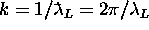is the wave number,is the unit vector along the propagation direction of the wave component, and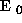is a complex vector perpendicular to. A laser beam is considered to be a superposition of plane waves with slightly differentand k. If the distribution ofaroundand that of k are Gaussian and if one ignores thedependence of, the laser field can be approximated by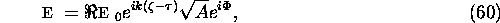where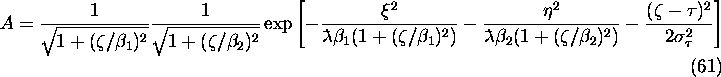where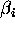(i=1,2) is the Rayleigh length   and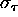is the r.m.s. pulse length.

The wave front is given by the contour of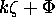. If one defines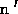by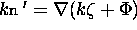,is nearly a unit vector and approximated by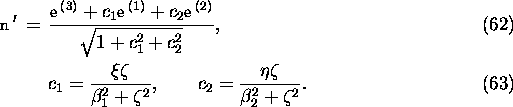In CAIN, when the relevant particle is at (t,x,y,s), or atin laser coordinate, the laser field is considered to be locally a plane wave with the power density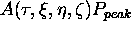, wave number k, and the propagation direction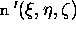.

There is some problem on the polarization because eq.(60) does not exactly satisfy the Maxwell equation. For simplicity, the basis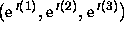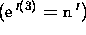for polarization is defined in the following manner: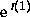is the unit vector along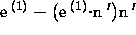and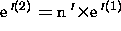. (This is irrelevant if only the longitudinal polarization is needed.)

The Lorentz transformation is a little complicated because eq.(60) is far from a covariant form. The particle coordinates and the external fields are transformed immediately when LORENTZ command is invoked and the transformation parameters are forgotten. In the case of lasers, the transformation is not done immediately but instead the transformation parameters are stored. When the laser is called at every time step for each particle, the particle coordinates are Lorentz transformed back to the frame where the laser was defined, and the calculated parameters (A,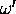,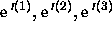) are transformed to the current Lorentz frame. Therefore, the Lorentz transformation is a little time-consuming.Next: Linear Compton Scattering Up: Laser Previous: Laser

Toshiaki Tauchi
Thu Dec 3 17:27:26 JST 1998